STAT 330: 98-1

Assignment 6 Solutions

1. Chapter 9 Q 54: Let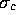be the population standard deviation for control rats and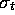be the treatment population standard deviation. Then to test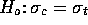against the one sided alternative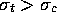we compute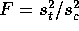and get upper tail P-values from F tables with 19 and 22 degrees of freedom. We get F = 2.85 and P just a bit bigger than 0.01 so that we conclude that the treatment population is more variable. (While the question asks a one-tailed question it is not entirely clear to me that the tail was not chosen after seeing the data; if so you should double the P-value. The conclusion is not really changed.)
2. Chapter 9 Q 56: The quantity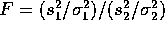has an F distribution so that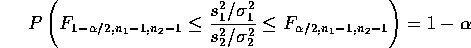The right hand inequality holds if and only if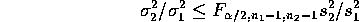Similarly, the first inequality can be rearranged to be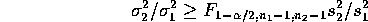so that the range between these two limits is a level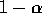confidence interval for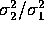. For the data we have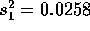and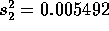. The critical point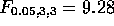while to find the lower tail critical point we use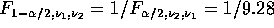. The interval is then 0.00549/(0.0258*9.28) to (9.28)(0.00549)/0.0258. Note that some people may have put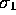on top.

3. Chapter 10 Q 10:

1.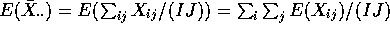. Use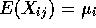and the fact that summing over j just multiplies by J to get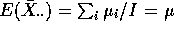.
2.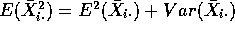. The variance of any average of J independent quantities each with variance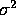is just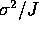so we get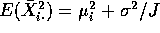.
3.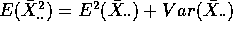which, using (a) and the rule in (b) is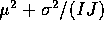.
4. We have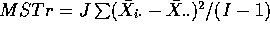. Expand out the square and use the fact that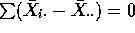to see that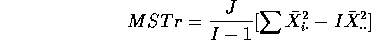Take expected values and put in the results of (b) and (c) to get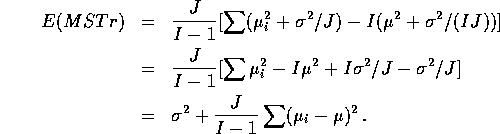5. Under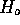the second of these terms is 0 so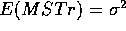while under the alternative the second term is positive so that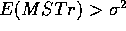.

4. Chapter 10 Q 42: For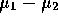we have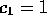,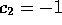, and all the other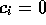so that the confidence interval foris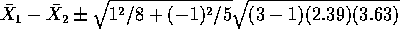(where we use the level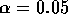for a 95% confidence interval). For the other intervals it is the values of the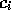which change. They are (1,0,-1), (0,1,-1) and (0.5,0.5,-1) respectively. Only the contrast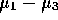is judged significantly different from 0. (Note the use of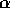not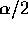; these are 95% confidence intervals.)
5. Chapter 10 Q 44: We have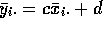and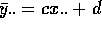. Subtracting we get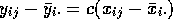so the the SSE for the y's is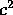times the SSE for the x's. Similarly we have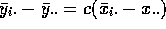so that the new SSTr istimes the old SSTr. The factorsthen cancel out in the formula for the F statistic so that the new F statistic is exactly equal to the old F statistic.
6. Chapter 10 Q46:

1. The two samples are now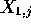for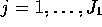and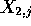for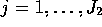. The two sample variances are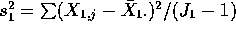and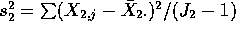. Then the pooled estimate ofis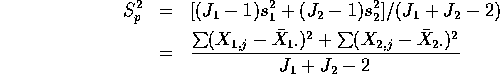which is just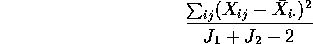which is in turn just the MSE.

2. The pooled statistic squared is just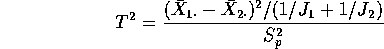Now write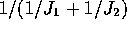as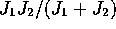to see that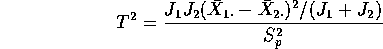Now examine the Treatment Sum of Squares. First note that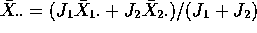. Use this to see that the Treatment Sum of Squares is given by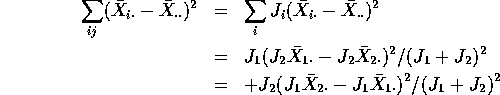which simplifies to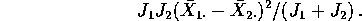This last is the numerator of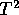.

Richard Lockhart
Fri Mar 6 10:16:01 PST 1998